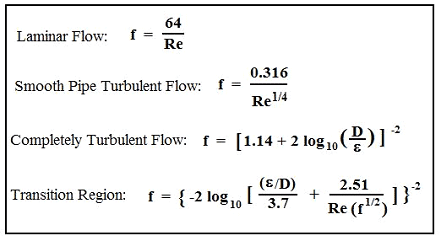Pipe Diameter Based on Friction Factor Calculator

Fluid Flow Design and Engineering

Calculator will determine pipe diameter required based on Head Loss, hL, and Frictional Pressure Drop, DPf, for given flow rate, Q, pipe diam., D, pipe length, L, pipe roughness, e, and fluid properties, r & m.

 Moody Friction Factor EquationPipe Roughness ValuesAll calculators require a Premium Membership NOTE: This iterative procedure doesn't converge smoothly to a solution. If the calculated pipe diameter in this step is larger than the assumed pipe diameter above, then replace the assumed pipe diameter value with the next larger standard pipe size. Repeat until you find the smallest standard pipe diameter that gives a smaller calculated required pipe diameter. That is your solution. With the example values given here, an assumed pipe diameter of 3 inches gives a calculated pipe diameter requirement of 3.4 inches, increasing the assumed pipe diameter to the next standard pipe size ( 3.5 inches ) gives a calculated pipe diameter requirement of 1.8 inches, so a 3.5 inch size is the minimum standard pipe diameter that will do the job.Membership Register | LoginHomeEngineering Book StoreEngineering ForumExcel App. DownloadsOnline Books & ManualsEngineering NewsEngineering VideosEngineering CalculatorsEngineering ToolboxGD&T Training Geometric Dimensioning TolerancingDFM DFA TrainingTraining Online EngineeringAdvertising CenterCopyright Notice